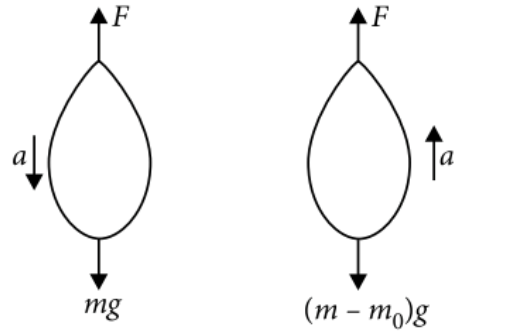Pseudo force
Question

# A balloon with mass m is descending down with an acceleration a (where  a < g).  How much mass should be removed from it so that it starts moving up with an acceleration a ?

Moderate
Solution

## Let F be the upthrust of the air. As the balloon is descending down with an acceleration a.Let mass ${m}_{0}$ be removed from the balloon so that it starts moving up with an acceleration a. Then,$\begin{array}{l}F-\left(m-{m}_{0}\right)g=\left(m-{m}_{0}\right)a\\ F-mg+{m}_{0}g=ma-{m}_{0}a\end{array}$                           . . . .(ii)$\begin{array}{l}{m}_{0}g=2ma-{m}_{0}a\\ {m}_{0}g+{m}_{0}a=2ma\\ {m}_{0}\left(g+a\right)=2ma\\ {m}_{0}=\frac{2ma}{g+a}\end{array}$

Get Instant Solutions